# Test: MCQs (One or More Correct Option): The s-Block Elements | JEE Advanced

## 23 Questions MCQ Test Chemistry 35 Years JEE Main & Advanced Past year Papers | Test: MCQs (One or More Correct Option): The s-Block Elements | JEE Advanced

Description
Attempt Test: MCQs (One or More Correct Option): The s-Block Elements | JEE Advanced | 23 questions in 50 minutes | Mock test for JEE preparation | Free important questions MCQ to study Chemistry 35 Years JEE Main & Advanced Past year Papers for JEE Exam | Download free PDF with solutions
QUESTION: 1

### A substance absorbs CO2 and voilently reacts with water.The substance is

Solution: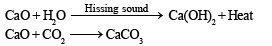QUESTION: 2

### HCl is added to following oxides. Which one would give H2O2?

Solution:

BaO2.8H2O + H2SO4 → BaSO4 + H2O2 + 8H2O

QUESTION: 3

### Calcium is obtained by

Solution:

Ca is obtained by electrolysis of molten mixture of CaCl2 mixed with CaF2.

QUESTION: 4

A solution of sodium metal in liquid ammonia is strongly reducing due to the presence of

Solution:

The free ammoniated electrons make the solution of Na in liquid NH3 a very powerful reducing agent.
NOTE : The ammonical solution of an alkali metal is rather favoured as a reducing agent than its aqueous solution because in aqueous solution the alkali metal being highly electropositive evolves hydrogen from water (thus H2O acts as an oxidisng agent) while its solution in ammonia is quite stable, provided no catalyst (transition metal) is present.

QUESTION: 5

Heavy water is

Solution:

Heavy water is D2O, deuterium oxide.

QUESTION: 6

The hydration energy of Mg++ is larger than that of :

Solution:

Na+ ion has larger size than Mg2+ ion and hence hydration energy of Mg2+ is larger than that of Na+.

QUESTION: 7

The oxide that gives hydrogen peroxide on treatment with a dilute acid is :

Solution:

Na2O2 + H2SO4 (20% ice cold) → Na2SO4 + H2O2

QUESTION: 8

Molecular formula of Glauber ’s salt is :

Solution:

Glauber ’s salt is Na2SO4.10 H2O.

QUESTION: 9

Hydrogen gas will not reduce :

Solution:

NOTE : The more electropositive metal will not be reduced by hydrogen.
Among given choices only Al is more electropositive than hydrogen.
∴ It will not be reduced by hydrogen.

QUESTION: 10

The pair of compounds which can not exist together in solution is :

Solution:

NOTE : Acidic and basic salts cannot exist together.
Since NaHCO3 is an acid salt of H2CO3, it reacts with NaOH to form Na2CO3 and H2O.
NaHCO3 + NaOH → Na2CO3 + H2O

QUESTION: 11

The metallic lustre exhibited by sodium is explained by

Solution:

Oscillation of loose electrons.

QUESTION: 12

The volume strength of 1.5 N H2O2 solution is

Solution:

Volume strength = Normality  × 5.6 = 1.5 × 5.6 = 8.4 L

QUESTION: 13

The following compounds have been arranged in order of their increasing thermal stabilities. Identify the correct order.

K2CO3 (I) MgCO3 (II)  CaCO3 (III) BeCO3 (IV)

Solution:

The increasing thermal stability is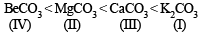NOTE : Increasing size of cation decreases its polarization ability towards carbonate, making the compound more stable.

QUESTION: 14

The set representing the correct order of first ionization potential is

Solution:

In going from top to bottom in a group, the first ionization potential decreases, thus
Be > Mg > Ca

QUESTION: 15

A sodium salt on treatment with MgCl2 gives white precipitate only on heating. The anion of the sodium salt is

Solution: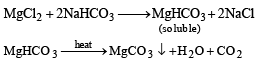QUESTION: 16

Hydrogen peroxide in its reaction with KIO4 and NH2OH respectively, is acting as a

Solution:

KIO4 + H2O2 → KIO3 + H2O + O2
Thus H2O2 is acting as a reducing agent 2NH2OH + H2O2 → N2 + 4H2O
Here H2O2 is acting as an oxidising agent

*Multiple options can be correct
QUESTION: 17

When zeolite, which is hydrated sodium aluminium silicate, is treated with hard water the sodium ions are exchanged with

Solution: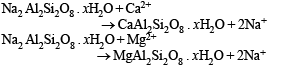*Multiple options can be correct
QUESTION: 18

The species that do not contain peroxide ions are

Solution:

Only H2O2 (hydrogen peroxide) and BaO2 (Barium peroxide) contain peroxide ions. So (a) and (c) are the correct choices.

*Multiple options can be correct
QUESTION: 19

Highly pure dilute solution of sodium in liquid ammonia

Solution:

A small piece of sodium is cut to expose a fresh surface. The sodium is dropped into liquid ammonia at a temperature of approximately -33 degrees Celsius. Some of the sodium dissolves, forming sodium cations surrounded by ammonia molecules and electrons surrounded by ammonia molecules. The solvated electrons give the blue color to the solution.
Because of the mobility of the electrons, the solution is a good electrical conductor. Bubbles of hydrogen gas are formed by a second reaction that also produces sodium amide. More concentrated solutions appear bronze-colored and have a conductivity similar to metals.
2Na+2NH​→ 2NaNH2+H2.
Hence options A,B,C & D are correct

*Multiple options can be correct
QUESTION: 20

The species present in solution when CO2 is dissolved in water are

Solution: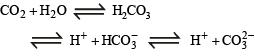*Multiple options can be correct
QUESTION: 21

MgSO4 on reaction with NH4OH and Na2HPO4 forms a white crystalline precipitate. What is its formula?

Solution: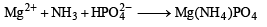*Multiple options can be correct
QUESTION: 22

The compound(s) formed upon combustion of sodium metal in excess air is (are)

Solution: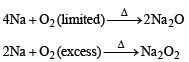*Multiple options can be correct
QUESTION: 23

The reagent(s) used for softening the temporary hardness of water is (are)

Solution:

Temporary hardness is due to bicarbonates of calcium and magnesium. Temporary hardness can be removed by Clark’s process, which involves the addition of slaked lime, Ca(OH)2. Washing soda (Na2CO3) removes both the temporary and permanent hardness by converting soluble calcium and magnesium compounds into insoluble carbonates.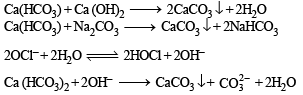Use Code STAYHOME200 and get INR 200 additional OFF Use Coupon Code